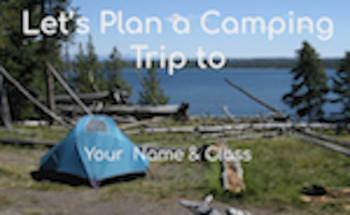# Plan A Camping TripSubject
Resource Type
File Type

PDF

(3 MB|15 pages)
Product Rating
4.0
(2 Ratings)
Standards
• Product Description
• StandardsNEW

Plan a camping trip with digital tools to support reasoning and problems solving. Let kids show what they know about ratios, surface area & volume, and adding and subtracting decimals.

This is a Google Slides Project-Based Activity that contains 6 different activities for students to apply concepts related to 5th & 6th grade math in a real-life context.

Determine the distance between two points

Students will design a tent (surface area & volume)

Calculate mileage (multiplying decimals)

Create a ratio table of mileage (ratio)

Design a camp site (area & perimeter)

Plan food cost ( adding and subtracting decimals)

Solve unit rate problems including those involving unit pricing and constant speed. For example, if it took 7 hours to mow 4 lawns, then at that rate, how many lawns could be mowed in 35 hours? At what rate were lawns being mowed?
Use ratio and rate reasoning to solve real-world and mathematical problems, e.g., by reasoning about tables of equivalent ratios, tape diagrams, double number line diagrams, or equations.
Understand the concept of a ratio and use ratio language to describe a ratio relationship between two quantities. For example, “The ratio of wings to beaks in the bird house at the zoo was 2:1, because for every 2 wings there was 1 beak.” “For every vote candidate A received, candidate C received nearly three votes.”
Represent three-dimensional figures using nets made up of rectangles and triangles, and use the nets to find the surface area of these figures. Apply these techniques in the context of solving real-world and mathematical problems.
Find the volume of a right rectangular prism with fractional edge lengths by packing it with unit cubes of the appropriate unit fraction edge lengths, and show that the volume is the same as would be found by multiplying the edge lengths of the prism. Apply the formulas 𝘝 = 𝘭 𝘸 𝘩 and 𝘝 = 𝘣 𝘩 to find volumes of right rectangular prisms with fractional edge lengths in the context of solving real-world and mathematical problems.
Total Pages
15 pages
N/A
Teaching Duration
N/A
Report this Resource to TpT
Reported resources will be reviewed by our team. Report this resource to let us know if this resource violates TpT’s content guidelines.